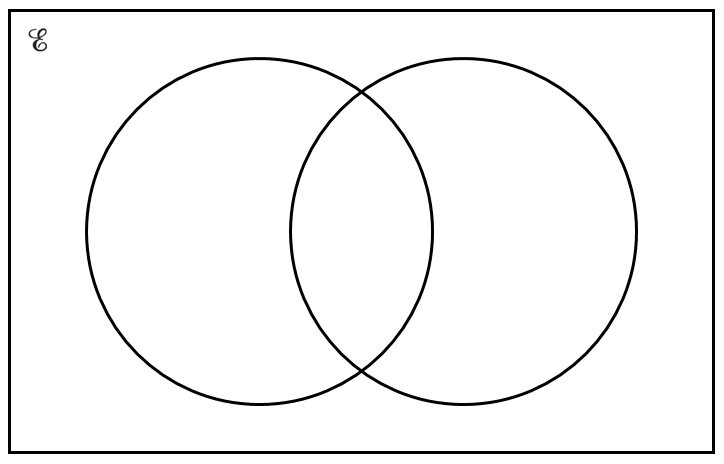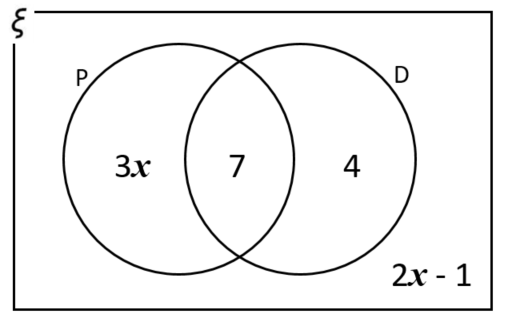# Exam-Style Questions.

## Problems adapted from questions set for previous Mathematics exams.

### 1.

GCSE Higher

If the universal set is the set of positive even numbers less than 30 and:

$$A = \{4, 8, 12, 16, 20, 24, 28\}$$ $$B = \{6, 12, 18, 24, 28\}$$

(a) Complete the Venn diagram with this information.A number is chosen at random from the universal set.

(b) What is the probability that the number is in the set $$A \cap B$$?

### 2.

GCSE Higher

(a) Complete the Venn diagram to represent this information:

$$\mathscr{E} = \{-4, -3, -2, -1, 0, 1, 2, 3, 4\}$$ $$A = \{1,2,3,4\}$$ $$B = \{-4,-2,2,4\}$$A number is chosen at random from the universal set $$\mathscr{E}$$.

(b) What is the probability that the number is in the set $$A \cap B$$?

### 3.

GCSE Higher

The Venn diagram represents a collection of 40 books on sale in an online store.

P represents the books available in paperback format.

D represents the books available in digital format.(a) One book from the collection is picked at random. Work out the probability that the book is available in digital format.

(b) One of the books that is available in paperback format is picked at random. Work out the probability that this book is also available in digital format.

If you would like space on the right of the question to write out the solution try this Thinning Feature. It will collapse the text into the left half of your screen but large diagrams will remain unchanged.

The exam-style questions appearing on this site are based on those set in previous examinations (or sample assessment papers for future examinations) by the major examination boards. The wording, diagrams and figures used in these questions have been changed from the originals so that students can have fresh, relevant problem solving practice even if they have previously worked through the related exam paper.

The solutions to the questions on this website are only available to those who have a Transum Subscription.

Exam-Style Questions Main Page

Search for exam-style questions containing a particular word or phrase:

To search the entire Transum website use the search box in the grey area below.